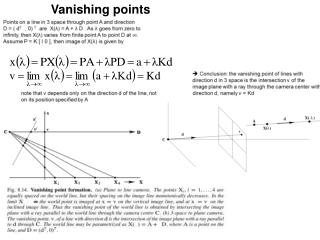DownloadDownload PresentationVanishing points

# Vanishing points

Download Presentation## Vanishing points

- - - - - - - - - - - - - - - - - - - - - - - - - - - E N D - - - - - - - - - - - - - - - - - - - - - - - - - - -
##### Presentation Transcript

1. Vanishing points

2. ML estimate of a vanishing point from imaged parallel scene lines • Estimate the VP and fitted lines simultaneously by solving a nonlinear • Levenson Marquardt optimization algorithm; • One option: • find pariwise intersection, then compute centrod of that intersection

3. Vanishing lines

4. If K is known, then plane vanishing line may be used to find information about the plane • Can show that plane with vanishing Line l has orientation KT l in camera’s Euclidean coordinate frame • Plane can be metrically rectified given only its vanishing line: • compute normal from vanishing line, then synthetically rotate • camera by homography so that the plane is fronto parallel, ie. • parallel to the image plane • If l1 and l2 are vanishing lines for two Planes, then the angle between the planes Is given by : ω θ ω ω

5. B c a Vanishing point of a line parallel to a plane lies on the vanishing line of the plane

6. Affine properties can be measured only from vanishing line of a plane and vanishing point of a direction not parallel to the plane Convenient to think of scene plane π as the horizontal ground plane; vanishing line is l is the horizon. Convenient to think of the direction orthogonal to the scene plane as vertical; v is the vertical vanishing point Given l and v, we can measure the relative length of two line segments in the vertical direction; No need to know K In general direction v need not be orthogonal to plane π

7. π Use the tiles on the floor (horizontal plane) to find the vanishing line of the plane. Use the vertical lines in the scene To determine the vertical vanishing point.

8. Computing K from scene and internal constraints

9. Calibration from vanishing points and lines Principal point is the orthocenter of the trinagle made of 3 orthogonol vanishing lines Assumes zero skew, square pixels and 3 orthogonal vanishing points

10. Assume zero skew , square pixels, and principal point is at the image center Then ω is diagonal{1/f^2, 1/f^2,1) i.e. one degree of freedom need one more Constraint to determine f, the focal length  two vanishing points corresponding To orthogonal directions. From two vanishing point, can also find vanishing line of the building façade; Given K and vanishing line l, synthetically rotate the camera so that façade is Fronto parallel: map the image with a homography

11. Scene consists of three dominant and mutually orthogonal planes: building façade on the right; on the left, and ground plane Parallels line sets in three orthogonal directions define three vanishing points and together with the constraint of square pixels of camera , calibration can be performed. From vanishing points find three vanishing lines for the 3 planes. Vanishing lines and ω can be used to find homography for each plane Use homography to texture map the appropriate image regions onto the orthogonal planes of the model

12. The calibrating conic: image of a cone with apex angle 45 degrees ; axis coinciding with the principal axis of the camera If camera matrix is P = K [ I 0 ] then map of the cone is a calibrating conic given by: